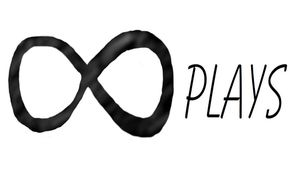By Bob

Here's an easy way to add text to odd shaped objects and make it conform to the shape of the object, basically it involves making a copy of the original object then clipping the text with the copy so it has the same shape as the main object. There's still a lot of work that needs to be done, but it works pretty good for the small amount of code it requires.

To try it out just copy and paste the code in the editor:

``````InputText = "Test";
Font = "Tlwg Typist:style=Bold Oblique";
Text_Size = 2.3; //[.1:.01:25]
Text_depth = 1;   //[1:.01:1.15]
TextSpacing = 1; //[.90:0.01:10]
Sphere_shape = 100; //[3:1:200]
Sphere_size= 10; //[10:.01:200]
Rotate_x=0; //[0:.01:360]
Rotate_y=0; //[0:.01:360]
Rotate_z=45; //[0:.01:360]
move_x =0; //[-200:.001:200]
move_y =0; //[-200:.001:200]
move_z =0; //[-200:.001:200]

rotate([-Rotate_x,Rotate_y,Rotate_z])
color([1,1,1])

intersection(){
translate([move_x,move_y,move_z])
rotate([90,0,0])
linear_extrude(Sphere_size,convexity = 11)
text(InputText, size=Text_Size, halign="center",  font=Font,spacing=TextSpacing,\$fn=Sphere_shape);
rotate([-Rotate_x,Rotate_y,Rotate_z])
scale([Text_depth,Text_depth,Text_depth])
sphere(Sphere_size/2,\$fn=Sphere_shape);
}

sphere(Sphere_size/2.01,\$fn=Sphere_shape);``````

This is an example using a sphere and adjusting the segments , I have also used an existing STL file by making some minor modifications.

Here I just added cylinders in place of the spheres:

And this one I imported an STL I made a few posts back, just to see how it would work with an odd shape:

Here is the code I used to add text to an existing STL file:

``````Path_to_STL_File ="/home/none3/Desktop/.stl";
InputText = "Test";
Font = "Tlwg Typist:style=Bold Oblique";
Text_Size = 5; //[.1:.01:25]
Text_depth =1.01; //[1.01:.01:1.15]
TextSpacing = 1; //[.90:0.01:10]
Text_smoothing = 100; //[3:1:200]
Extrude_depth= 50; //[10:.01:400]
Rotate_x=0; //[-360:.01:360]
Rotate_y=0; //[-360:.01:360]
Rotate_z=0; //[-360:.01:360]
move_x =0; //[-200:.001:200]
move_y =0; //[-200:.001:200]
move_z =0; //[-200:.001:200]

rotate([-Rotate_x,Rotate_y,Rotate_z])
color([1,1,1])

intersection(){
translate([move_x,move_y,move_z])
rotate([90,0,0])
linear_extrude(Extrude_depth,convexity = 11)
text(InputText, size=Text_Size, halign="center", font=Font,spacing=TextSpacing,\$fn=Text_smoothing);
rotate([0,0,90])
scale([Text_depth,Text_depth,Text_depth])
import(Path_to_STL_File,convexity=11);

}
rotate([0,0,90])
import(Path_to_STL_File,convexity=11);``````

There are a few limitations to overcome like wrapping the text around the entire object and making the text tilt-able so it can be fine tuned, but for most labeling jobs on odd surfaces this seems to work pretty well.

## Related ArticlesFollow